# 当一个程序员决定穿上粉裤子

08/24 00:29## 01.图像分割

• Mateusz Dziemian 提供的 segformer_b2_clothes 模型

• Valentina Feruere 提供的 YOLOS-Fashionpedia 模型

• Patrick John Chia 提供的 Fashion-CLIP 模型

• torch用于提取图像特征

• 来自 transformerssegformer

• 来自 torchvisionResizemasks_to_boxescrop

import torch
from torch import nn, tensor
from transformers import AutoFeatureExtractor, SegformerForSemanticSegmentation
import matplotlib.pyplot as plt
from torchvision.transforms import Resize
import torchvision.transforms as T
from torchvision.transforms.functional import crop


### 使用 Hugging Face 生成图像分割掩膜

get_segmentation函数需要三个参数：特征提取器（feature extractor）、模型（model）和图像（image）。

def get_segmentation(extractor, model, image):
inputs = extractor(images=image, return_tensors="pt")

outputs = model(**inputs)
logits = outputs.logits.cpu()

upsampled_logits = nn.functional.interpolate(
logits,
size=image.size[::-1],
mode="bilinear",
align_corners=False,
)

pred_seg = upsampled_logits.argmax(dim=1)
return pred_seg


upsampled_logits中的图像如下所示：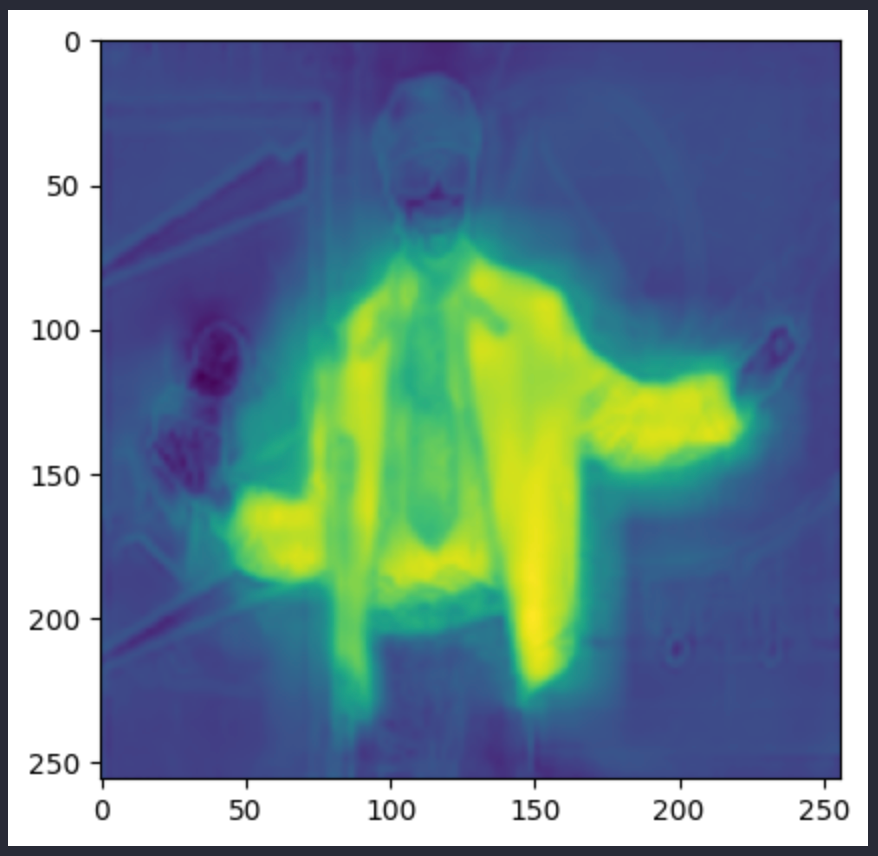pred_seg图像如下所示。上面两张都是 Andre 3000 的照片，但其实是不同的图像：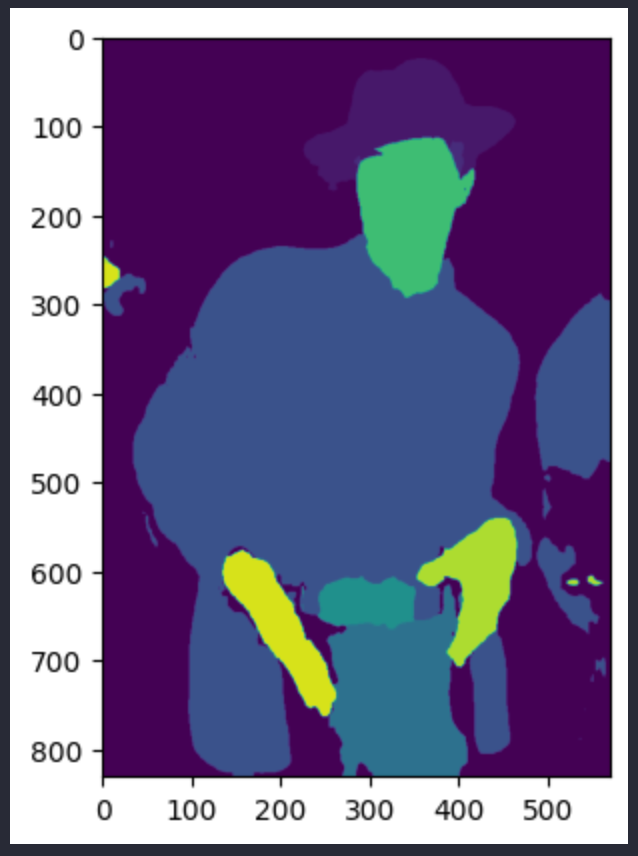# 返回 2 个 lists masks (tensor) 和obj_ids(int)
# 来自 hugging face 的 "mattmdjaga/segformer_b2_clothes" 模型
obj_ids = torch.unique(segmentation)
obj_ids = obj_ids[1:]
masks = segmentation == obj_ids[:, None, None]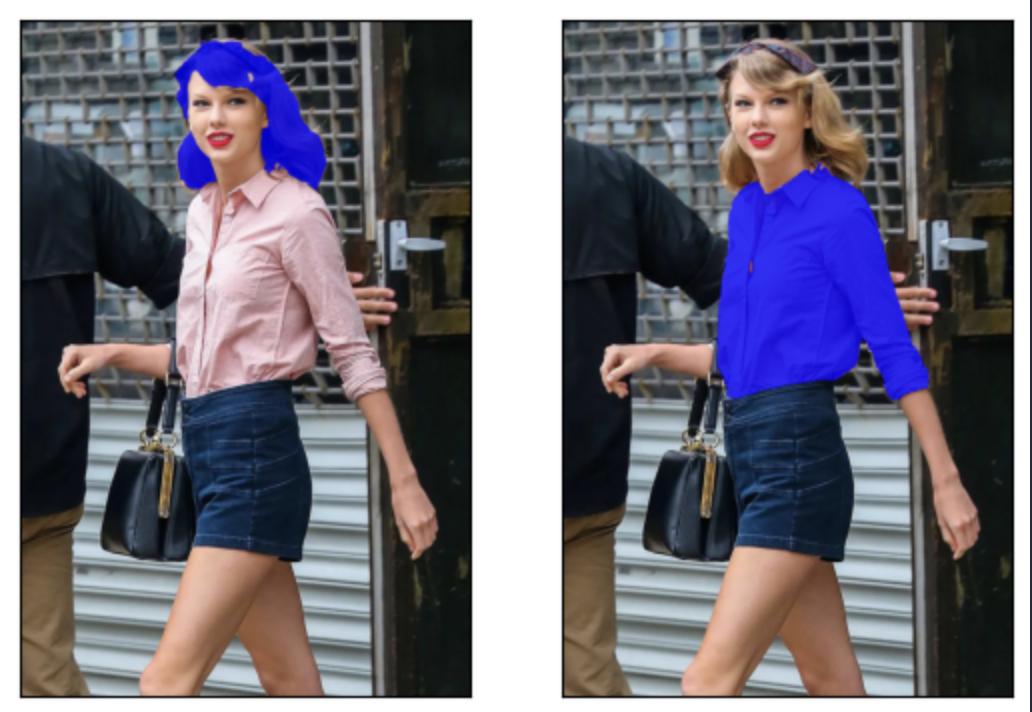### 使用 Pytorch 裁剪和调整图像大小

def crop_images(masks, obj_ids, img):
crop_boxes = []
for box in boxes:
crop_box = tensor([box, box, box-box, box-box])
crop_boxes.append(crop_box)

preprocess = T.Compose([
T.Resize(size=(256, 256)),
T.ToTensor()
])

cropped_images = {}
for i in range(len(crop_boxes)):
crop_box = crop_boxes[i]
cropped = crop(img, crop_box.item(), crop_box.item(), crop_box.item(), crop_box.item())
cropped_images[obj_ids[i].item()] = preprocess(cropped)
return cropped_images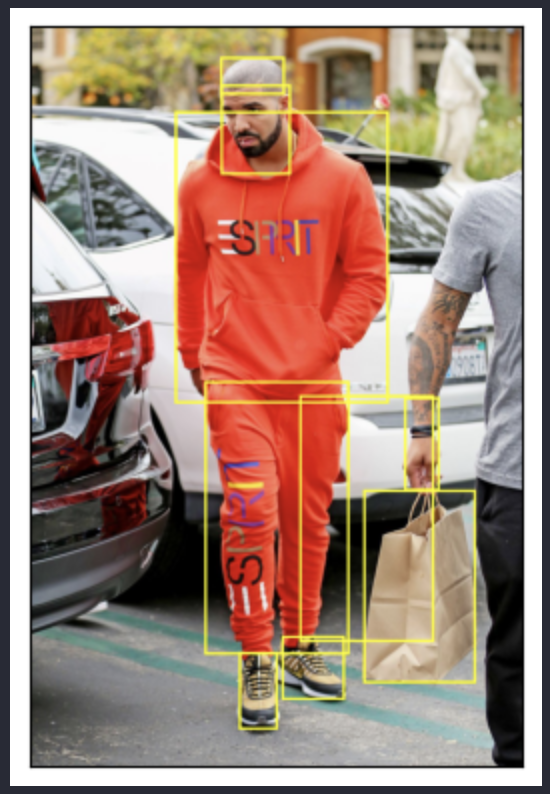## 02.将图像数据添加至向量数据库中

from milvus import default_server
from pymilvus import utility, connections
default_server.start()
connections.connect(host="127.0.0.1", port=default_server.listen_port)

DIMENSION = 2048
BATCH_SIZE = 128
COLLECTION_NAME = "fashion"
TOP_K = 3

# 如果遇到 SSL 证书 URL 错误，请在导入 resnet50 模型前运行此内容
import ssl
ssl._create_default_https_context = ssl._create_unverified_context


### 在向量数据库中定制 Schema 并存储元数据

Collection 创建完成后，构建索引。索引参数十分简单。选择 IVF Flat 的索引类型和 L2 相似度类型。这个索引是针对于 Collection 中的 embedding 向量字段。索引构建完成后，将 Collection 加载到内存中，以便后续操作。

from pymilvus import FieldSchema, CollectionSchema, Collection, DataType

fields = [
FieldSchema(name="id", dtype=DataType.INT64, is_primary=True, auto_id=True),
FieldSchema(name='filepath', dtype=DataType.VARCHAR, max_length=200),
FieldSchema(name="name", dtype=DataType.VARCHAR, max_length=200),
FieldSchema(name="seg_id", dtype=DataType.INT64),
FieldSchema(name='embedding', dtype=DataType.FLOAT_VECTOR, dim=DIMENSION)
]

schema = CollectionSchema(fields=fields)
collection = Collection(name=COLLECTION_NAME, schema=schema)

index_params = {
"index_type": "IVF_FLAT",
"metric_type": "L2",
"params": {"nlist": 128},
}
collection.create_index(field_name="embedding", index_params=index_params)


### 从 Nvidia ResNet50 模型获取 embedding 向量

# 加载 embedding 模型并删除最后一层输出
embeddings_model = torch.nn.Sequential(*(list(embeddings_model.children())[:-1]))
embeddings_model.eval()


def embed_insert(data, collection, model):
print(len(data))
print(data.size())
output = model(torch.stack(data)).squeeze()
print(type(output))
print(len(output))
print(len(output))
print(output)

collection.insert([data, data, data, output.tolist()])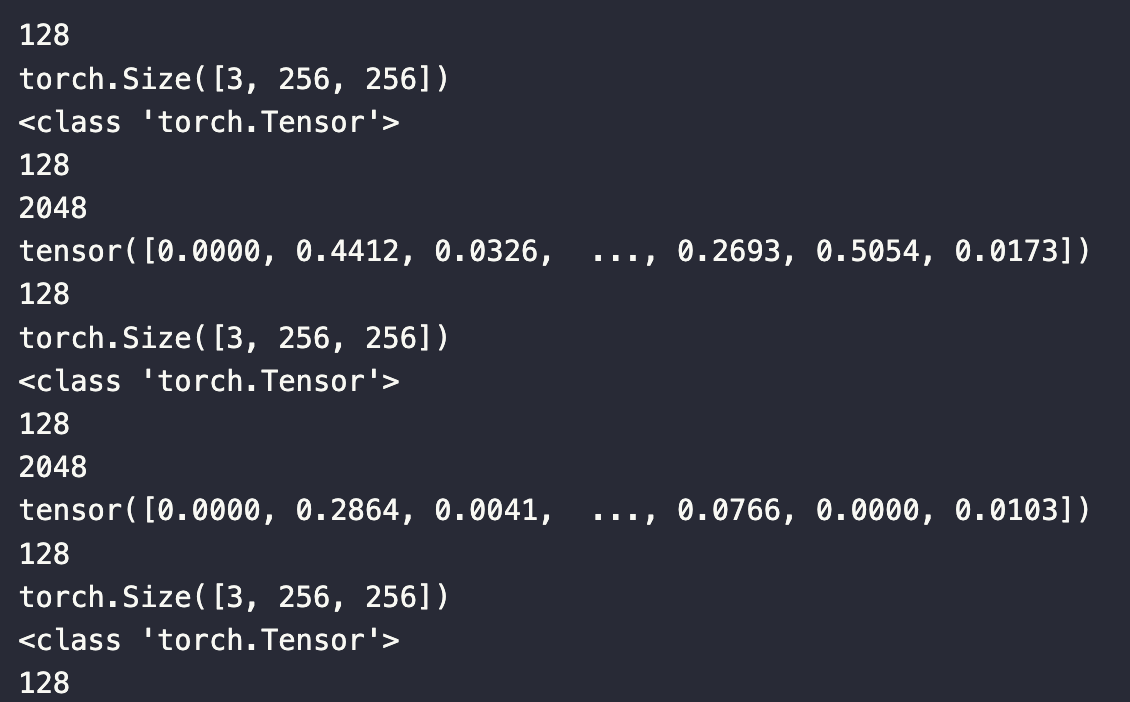### 将图像数据存储到向量数据库中

extractor = AutoFeatureExtractor.from_pretrained("mattmdjaga/segformer_b2_clothes")
model = SegformerForSemanticSegmentation.from_pretrained("mattmdjaga/segformer_b2_clothes")
import os
image_paths = []
for celeb in os.listdir("./photos"):
for image in os.listdir(f"./photos/{celeb}/"):
# print(image)
image_paths.append(f"./photos/{celeb}/{image}")


Milvus 期望输入格式为列表。在本项目中，我们使用了 4 个列表，分别对应图像、文件路径、名称和分割 ID。在 embed_insert 函数中，将图像转换为 embedding 向量。然后，循环遍历每个图像文件的文件路径，收集它们的分割 mask 并对其进行裁剪。最后，将图像及元数据添加到数据批处理中。

from PIL import Image
data_batch = [[], [], [], []]

for path in image_paths:
image = Image.open(path)
path_split = path.split("/")
name = " ".join(path_split.split("_"))
segmentation = get_segmentation(extractor, model, image)

for key, image in cropped_images.items():
data_batch.append(image)
data_batch.append(path)
data_batch.append(name)
data_batch.append(key)

if len(data_batch) % BATCH_SIZE == 0:
embed_insert(data_batch, collection, embeddings_model)
data_batch = [[], [], [], []]

if len(data_batch) != 0:
embed_insert(data_batch, collection, embeddings_model)

collection.flush()


## 03.寻找与你时尚风格最相似的明星

### 将上传图像转化为向量

def embed_search_images(data, model):
print(len(data))
print(data.size())
output = model(torch.stack(data))
print(type(output))
print(len(output))
print(len(output))
print(output)
if len(output) > 1:
return output.squeeze().tolist()
Else: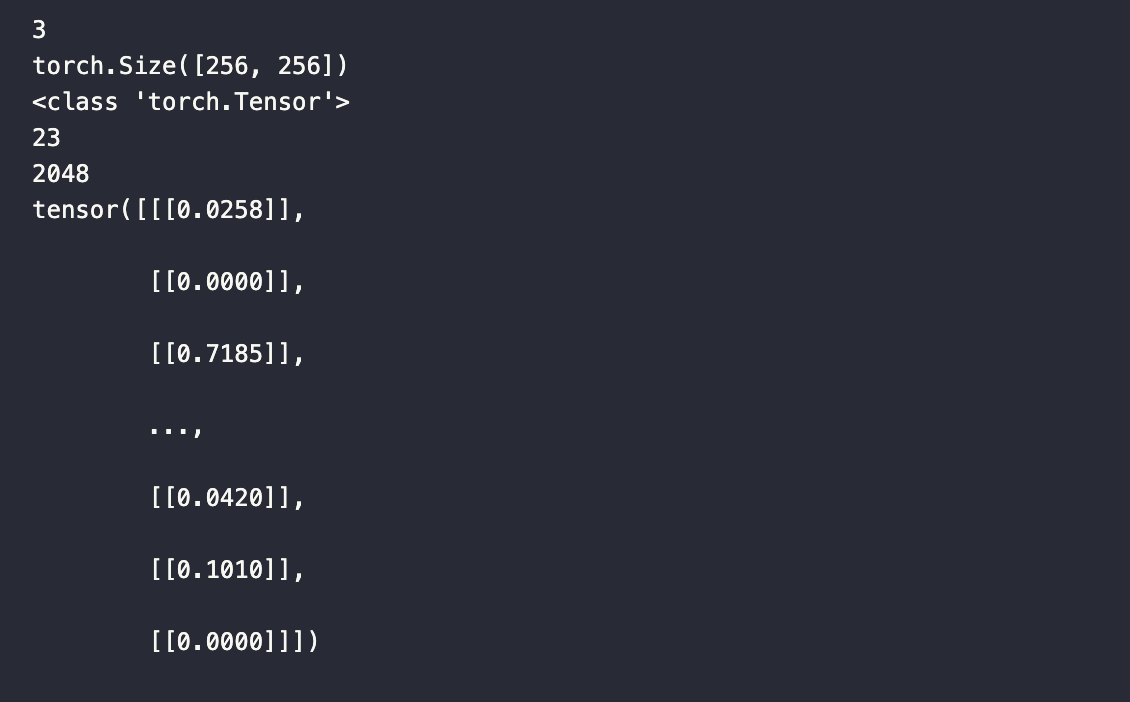# data_batch is a list of tensors
# data_batch is a list of filepaths to the images (string)
# data_batch is a list of the names of the people in the images (string)
# data_batch is a list of segmentation keys (int)
data_batch = [[], [], [], []]

search_paths = ["./photos/Taylor_Swift/Taylor_Swift_3.jpg", "./photos/Taylor_Swift/Taylor_Swift_8.jpg"]

for path in search_paths:
image = Image.open(path)
path_split = path.split("/")
name = " ".join(path_split.split("_"))
segmentation = get_segmentation(extractor, model, image)
for key, image in cropped_images.items():
data_batch.append(image)
data_batch.append(path)
data_batch.append(name)
data_batch.append(key)

embeds = embed_search_images(data_batch, embeddings_model)


### 查询向量数据库

import time
start = time.time()
res = collection.search(embeds,
anns_field='embedding',
param={"metric_type": "L2",
"params": {"nprobe": 10}},
limit=TOP_K,
output_fields=['filepath'])
finish = time.time()
print(finish - start)


for index, result in enumerate(res):
print(index)
print(result)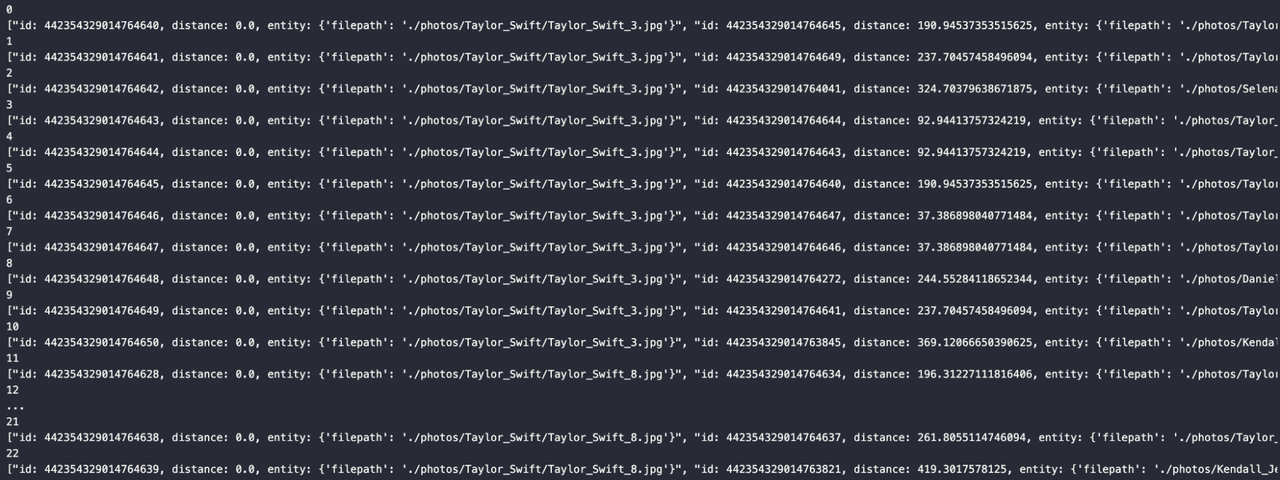🌟「寻找 AIGC 时代的 CVP 实践之星」 专题活动即将启动！

Zilliz 将联合国内头部大模型厂商一同甄选应用场景， 由双方提供向量数据库与大模型顶级技术专家为用户赋能，一同打磨应用，提升落地效果，赋能业务本身。0 评论
0 收藏
0